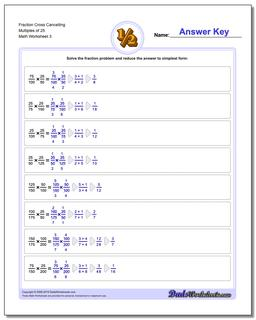# Math Worksheets: Fraction Multiplication: Fraction Multiplication: Fraction Cross Cancelling Multiples of 25 (Third Worksheet)## Fraction Cross Cancelling Multiples of 25 (Third Worksheet)

PropertyValue
DescriptionFraction Cross Cancelling Multiples of 25: The multiplying fraction worksheets in this section have a heavy emphasis on problems that require you to cross multiply (or cross cancel) to get the product. (Third Worksheet)
Resource TypeWorksheet